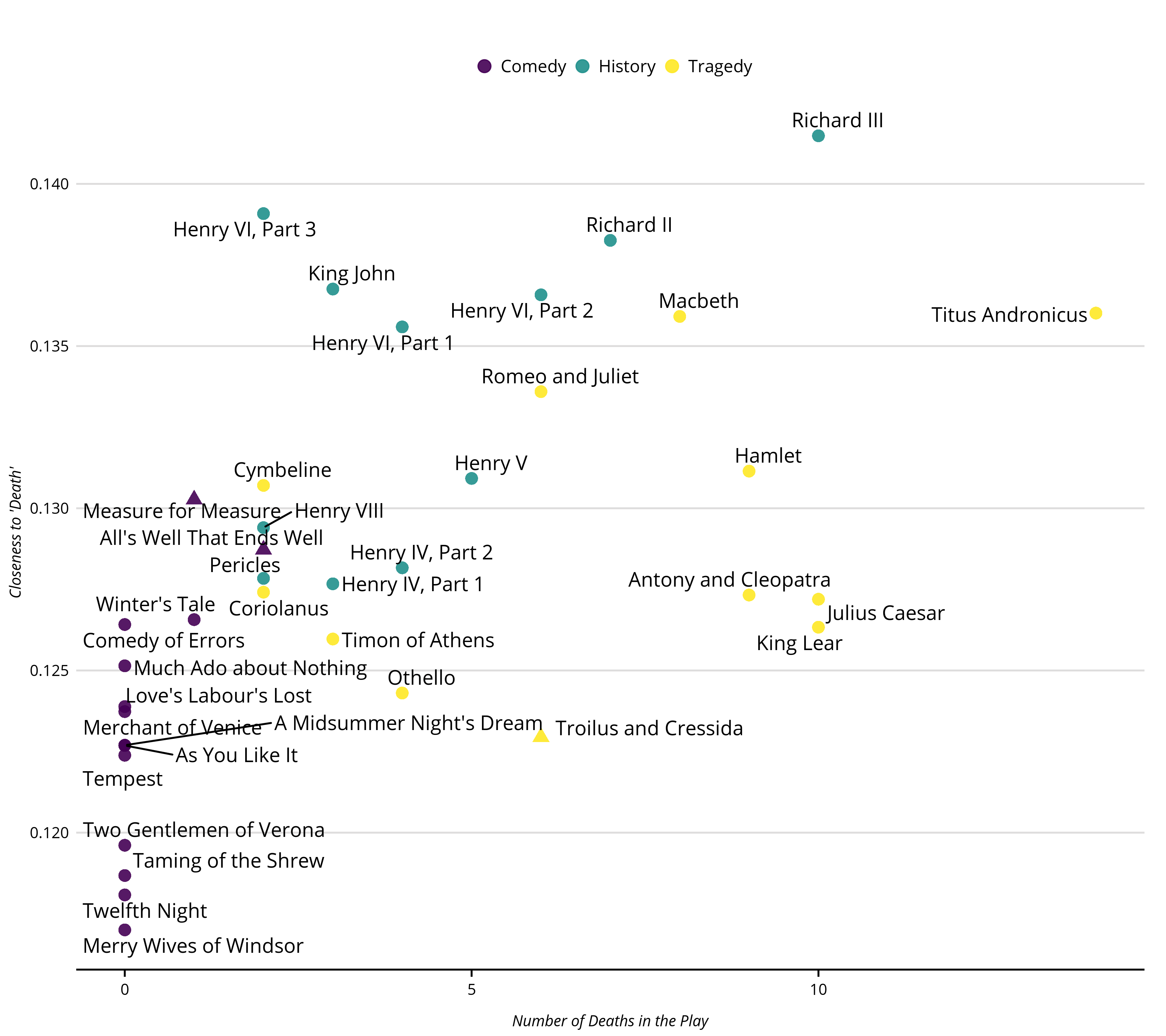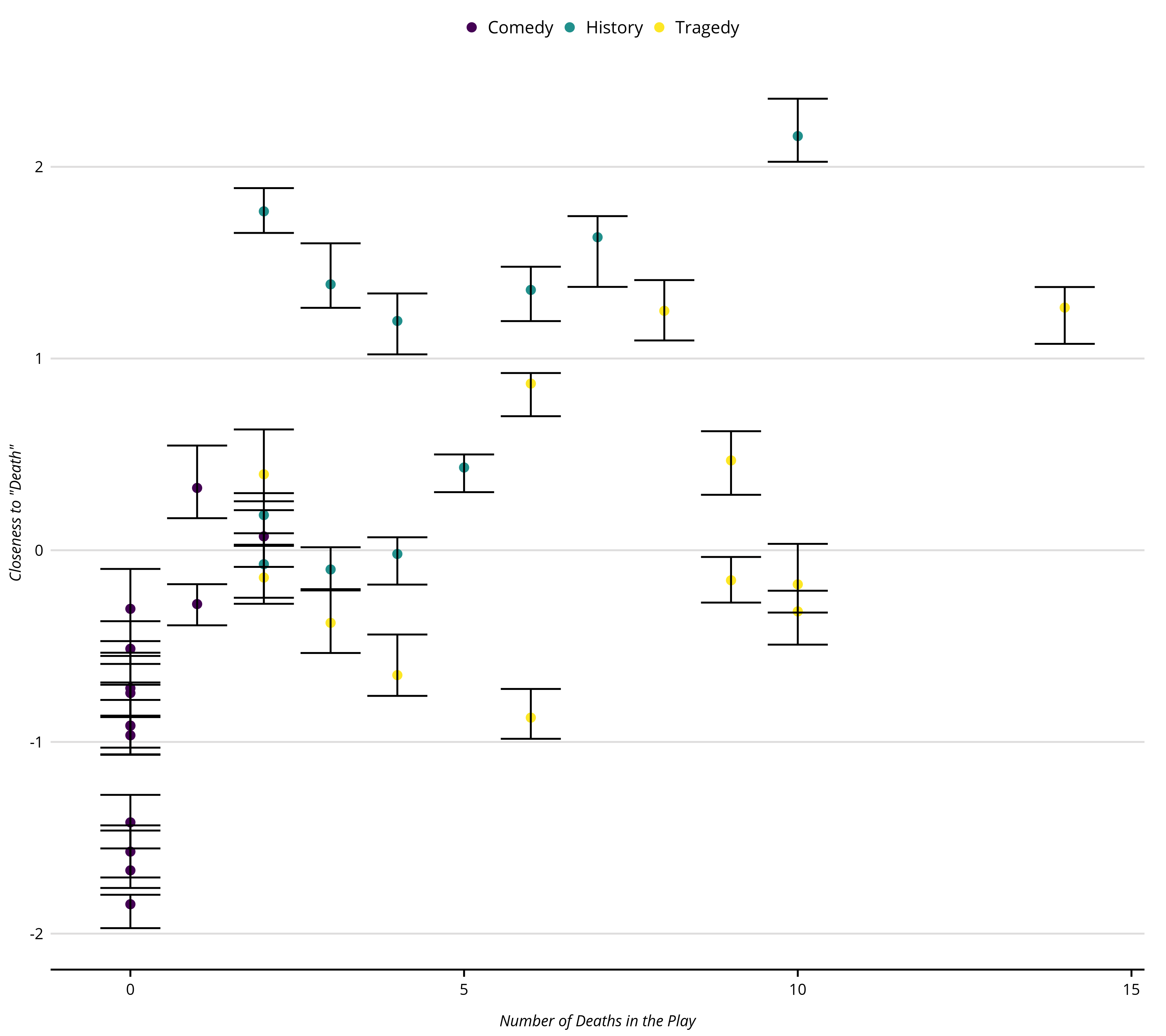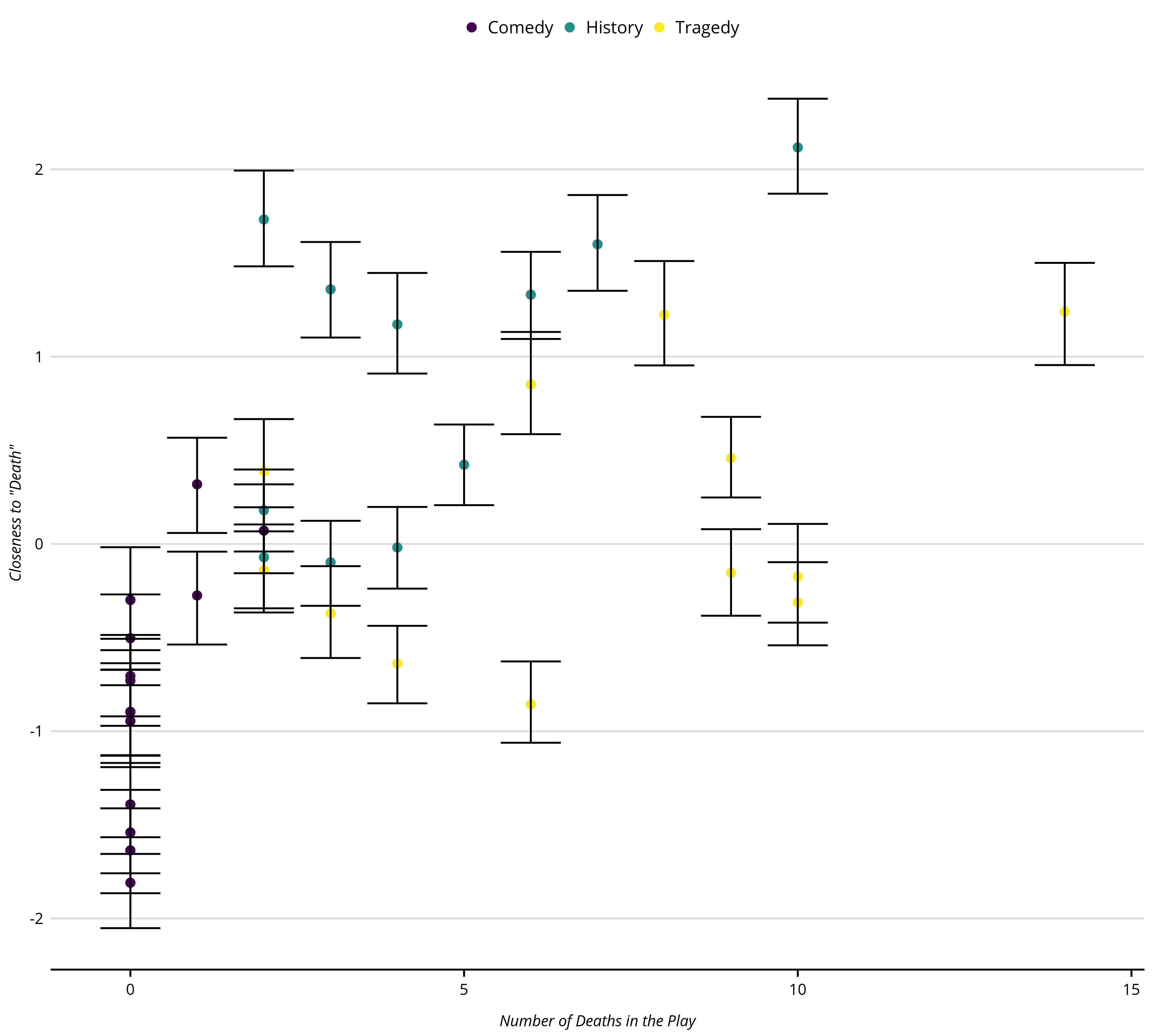pacman::p_load(textclean,
gutenbergr,
stringi,
ggrepel,
tidyverse,
text2map,
install = TRUE)

# optional
# devtools::install_gitlab("culturalcartography/text2map.theme")
text2map.theme::set_theme()
data("meta_shakespeare")

# Grab the text from Project GUTENBERG
df_text <- meta_shakespeare |>
select(gutenberg_id) |>
group_by(gutenberg_id) |>
summarize(text = paste(text, collapse = ", "))

Next we will take care of a bit of cleaning. Make sure there are no special characters, remove punctuation, take care of curly quotes (which can be a pain), and then squash contractions (rather than replacing apostrophes with a space, leaving a bunch of single letters). Finally, we will remove any digits that might be floating around (this is downloaded from the Internet after all). Then, make sure there is only a single space between and around words.

df_text <- df_text |> mutate(
## transliterate and lowercase
text = stri_trans_general(text, id = "Any-Latin; Latin-ASCII"),
text = tolower(text),
## punctuation
text = replace_curly_quote(text),
text = gsub("(\\w+[_'-]+\\w+)|[[:punct:]]+", "\\1", text),
text = replace_contraction(text),
## remove numbers
text = gsub('[[:digit:]]+', " ", text),
## take care of extra spaces
text = gsub("[[:space:]]+", " ", text),
text = trimws(text, whitespace = "[\\h\\v]")
)

Finally, we will need to convert the cleaned text of the plays into a Document-Term Matrix. The text2map package has a very efficient unigram DTM builder called dtm_builder() – it has very few options, but the unigram DTM is typically all we need.

dtm <- df_text |>
dtm_builder(text, gutenberg_id)
# text2map's handy DTM stats function
# let's just look at the basics
df_stats <- dtm_stats(dtm,
richness = FALSE,
distribution = FALSE,
central = FALSE,
character = FALSE
)

knitr::kable(df_stats, row.names = TRUE)
Measure Value
1 Total Docs 37
2 Percent Sparse 87.70%
3 Total Types 29957
4 Total Tokens 889429
5 Object Size 3.6 Mb

### Word Embeddings

You will need to load pre-trained word embeddings. We use the fastText English embeddings. We have also hosted an Rds file of these embeddings on Google Drive that you can download directly into your R session (it will take a couple minutes).

library(googledrive)
temp <- tempfile()
drive_download(as_id("17H4GOGedeGo0urQdDC-4e5qWQMeWLpGG"), path = temp, overwrite = TRUE)
ft_wv <- readRDS(temp)

### Concept Mover’s Distance

First, we will measure each play’s (defined by the DTM) closeness to “death” using CMDist.

# Run CMD for concept words and semantic direction
cmd_plays <- dtm |>
CMDist(cw = "death", # concept word
wv = ft_wv, # word embeddings
scale = FALSE)

Attach each CMD output to it’s respective play, using the gutetenberg_id to join.

df <- cmd_plays |>
rename(cmd_death = death) |>
mutate(doc_id = as.numeric(doc_id) ) |>
left_join(meta_shakespeare, by = c("doc_id" = "gutenberg_id")) |>
as_tibble()

Let’s quickly plot closeness to “death” by a count of actual deaths in the play. This is roughly the same plot we included in our paper.

p_play <- df |>
mutate(genre = as.factor(genre),
shape = factor(boas_problem_plays,
levels = c(0, 1),
labels = c("", "Problem Play"))) |>
ggplot(aes(x = body_count, y = cmd_death)) +
geom_point(aes(color = genre, shape = shape), size = 3,  alpha = .9) +
geom_text_repel(aes(label = short_title)) +
scale_size(guide = "none") +
labs(x = "Number of Deaths in the Play", y = "Closeness to 'Death'",
title = NULL,
color = "Genre",
shape = "Boas' Problem Play",
size = FALSE) +
guides(shape = FALSE)### Sensitivity Intervals

If you recall, we did a little preprocessing at the beginning – and typically analyst will do even more preprocessing. Recall, as well, that in a DTM each document is represented as a distribution of word counts. How “sensitive” is each estimate to a given distribution of words? We can test this sensitivity by resampling (with replacement) from each document’s word distribution. We do this by setting the sens_interval=TRUES option in the CMDist() function – underneath the hood, the CMDist() function is using dtm_resampler() to estimate new DTMs and re-running CMD on each, before reporting a given interval (default = c(0.025, 0.975)). We will use the default settings for now.

# Run CMD with sensitivity intervals
sens_plays <- CMDist(dtm = dtm,
cw = "death", # concept word
wv = ft_wv, # word embeddings
sens_interval = TRUE,
scale = TRUE)

df_sens <- sens_plays |>
rename(cmd_death = death) |>
mutate(doc_id = as.numeric(doc_id) ) |>
left_join(meta_shakespeare, by = c("doc_id" = "gutenberg_id")) |>
as_tibble()

Now, we can plot the upper and lower bounds obtained from the resampled DTMs, along with the point estimate from the original DTM.

p_play_sens <- df_sens |>
ggplot(aes(x = body_count, y = cmd_death)) +
geom_point(aes(color = as.factor(genre)), size = 2) +
geom_errorbar(aes(x = body_count, y = cmd_death,
ymin = death_lower,
ymax = death_upper),
color = "#000000") +
xlab("Number of Deaths in the Play") +
ylab('Closeness to "Death"')There are two optional parameters: alpha, and n_iters. The first designates what proportion of the words should be resampled. The default is 1, which means to resample the same number of words as the original DTM row, whereas 0.5 would be to resample half the number of words for each row, and 1.5 would be one and half times more words than the original row. The default for n_iters is the number of DTMs to resample. The default is 20.

# Run CMD with sensitivity intervals
play_sens_100 <- CMDist(
dtm = dtm,
cw = "death", # concept word
wv = ft_wv, # word embeddings
sens_interval = TRUE,
alpha = 0.5,
n_iters = 100L,
scale = TRUE)

play_sens_100 <- play_sens_100L |>
rename(cmd_death = death) |>
mutate(doc_id = as.numeric(doc_id) ) |>
left_join(meta_shakespeare, by = c("doc_id" = "gutenberg_id")) |>
as_tibble()

Re-plot the estimates with sensitivity intervals from the new estimates using 100 resampled DTMs with rows half the size of the original DTM. Again, recall the point estimate are always from the original DTM – it is not an average across the sampled DTMs. Only the bounds are generated from the sampled DTMs.

p_play_sens_100 <- play_sens_100 |>
ggplot(aes(x = body_count, y = death)) +
geom_point(aes(color = as.factor(genre)), size = 2) +
geom_errorbar(aes(x = body_count, y = death,
ymin = death_lower,
ymax = death_upper), color = "#000000") +
xlab("Number of Deaths in the Play") +
ylab('Closeness to "Death"')